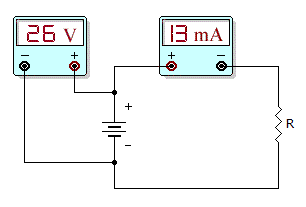# Electronics - Ohm's Law

### Exercise :: Ohm's Law - General Questions

6.

What is the kilowatt-hour consumption of a 40 W lamp if it remains on for 1750 h?

 A. 43.75 B. 70 C. 43,750 D. 70,000

Answer: Option B

Explanation:

(1,750 x 40)/1000 = 70 kilowatt-hour.

7.

A damaged resistor

 A. may appear burned B. may have an increased resistance value C. may be checked with an ohmmeter D. All of the above

Answer: Option D

Explanation:

No answer description available for this question. Let us discuss.

8.

Power supplies differ from batteries in that

 A. power supply output voltages are ac and not dc B. power supply voltages are not stable C. power supplies are low-current devices D. None of the above

Answer: Option D

Explanation:

No answer description available for this question. Let us discuss.

9.What is the resistor value in the given circuit?

 A. 200B. 1 kC. 2 kD. 4 kAnswer: Option C

Explanation:

No answer description available for this question. Let us discuss.

10.

If voltage across a resistance doubles

 A. the current is halved B. the resistance doubles C. the current is unchanged D. none of the above

Answer: Option D

Explanation:

No answer description available for this question. Let us discuss.

#### Current Affairs 2021

Interview Questions and Answers